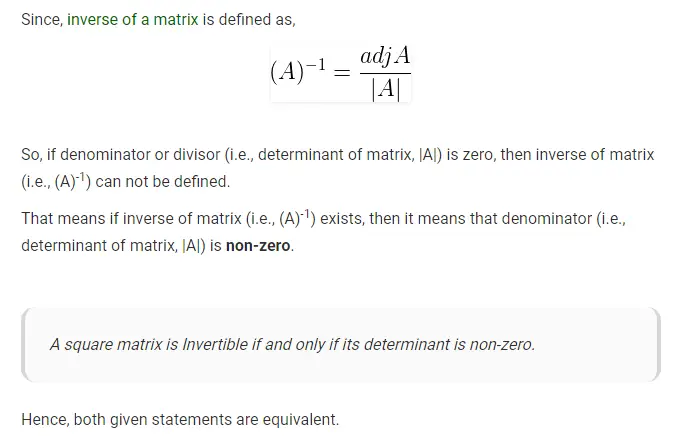# Gate CS-2019 Question Paper With Solutions

Q. 16 Let X be a square matrix. Consider the following two statements on X.

```I. X is invertible
II. Determinant of X is non-zero```

Which one of the following is TRUE?

(A) I implies II; II does not imply I

(B) II implies I; I does not imply II

(C) I does not imply II; II does not imply I

(D) I and II are equivalent statements